# A Novel Hybrid Spotted Hyena Optimizer: An Algorithm for Fuel Consumption Capacitated Vehicle Routing Problem

Title: A Novel Hybrid Spotted Hyena Optimizer: An Algorithm for Fuel Consumption Capacitated Vehicle Routing Problem
Dana Marsetiya Utama, Aminatul Yurifah, Annisa Kesy Garside

Corresponding email:

Utama, D.M., Yurifah, A., Garside, A.K., 2023. A Novel Hybrid Spotted Hyena Optimizer: An Algorithm for Fuel Consumption Capacitated Vehicle Routing Problem. International Journal of Technology. Volume 14(5), pp. 1049-1059

172
 Dana Marsetiya Utama Department of Industrial Engineering, University of Muhammadiyah Malang, Jl. Tlogomas No. 246, 65144 Malang, East Java, Indonesia Aminatul Yurifah Department of Industrial Engineering, University of Muhammadiyah Malang, Jl. Tlogomas No. 246, 65144 Malang, East Java, Indonesia Annisa Kesy Garside Department of Industrial Engineering, University of Muhammadiyah Malang, Jl. Tlogomas No. 246, 65144 Malang, East Java, Indonesia
Email to Corresponding Author

Abstract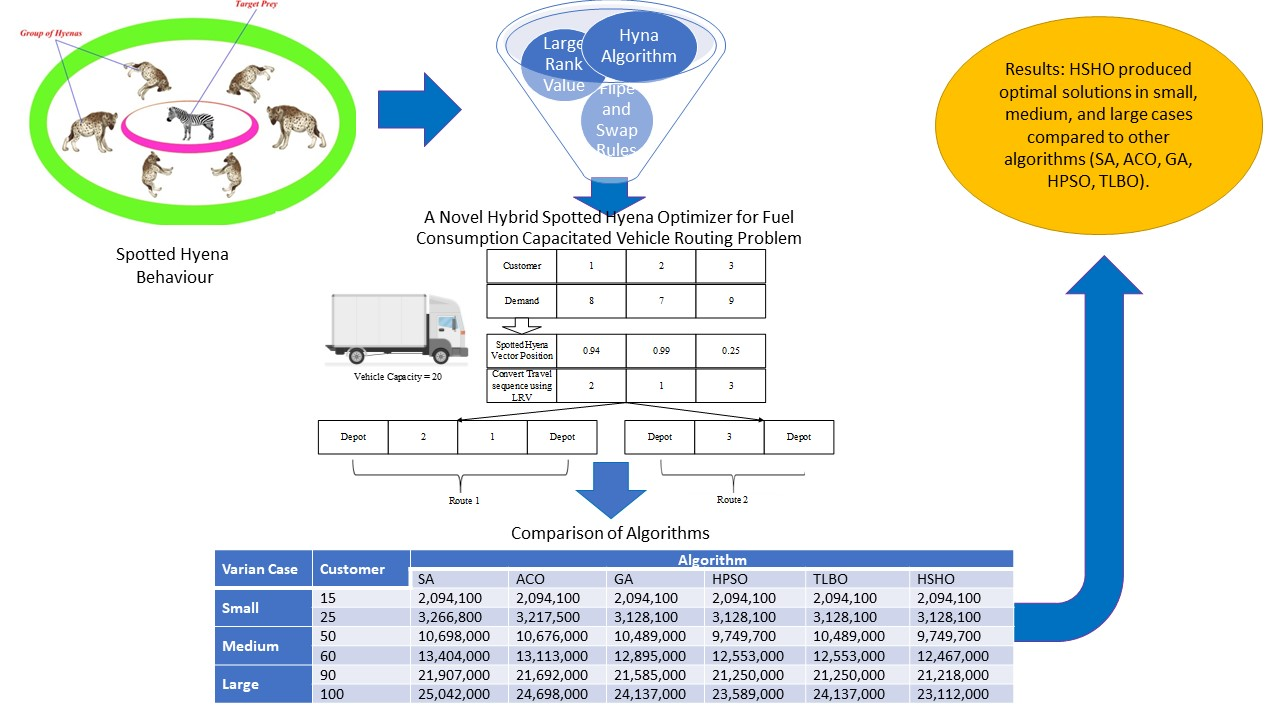Distribution activities are closely related to the objective function of minimizing fuel consumption, which is affected by distance and product load in transportation. This indicates the need for optimization to improve company performance. Therefore, this study aims to develop a new Hybrid Spotted Hyena Optimizer (HSHO) algorithm, to minimize the total transportation and fuel costs. This was provided by applying the Large Rank Value (LRV) procedure to convert hyena positions to travel sequences. This also proposed a Flip and Swap rule in each iteration to improve the algorithm's performance. Furthermore, a mathematical model was developed for the Fuel Consumption Capacitated Vehicle Routing Problem (FCCVRP) by considering the load and FC (fuel consumption) rates between the nodes. This indicated that several population variations, iterations, and several nodes were used to investigate the effectiveness of the HSHO algorithm. The results showed increased population parameters, and HSHO iterations reduced the FCCVRP total transportation costs. Furthermore, decreasing the fuel consumption rate between nodes affected reduced fuel consumption. In addition, the proposed HSHO produced a more optimal total transportation cost than the state-of-the-art algorithm.

Capacitated vehicle routing problem; Distribution; Fuel consumption; Spotted hyena optimizer

Introduction

The supply chain reportedly plays a vital role in organizational performance (Abdulameer, Yaacob, and Ibrahim, 2020; Ibrahim et al. 2020), through some activities such as procurement (Deepradit et al., 2020), production scheduling (Utama et al., 2019), and transportation (Sitompul and Horas, 2021; Benyamin, Farhad, and Saeid, 2021), where fuel consumption is found to be a crucial factor (Ozener and Ozkan, 2020; Norouzi, Sadegh-Amalnick, and Tavakkoli-Moghaddam, 2017). According to Sahin et al. (2009), a road transportation company in Shanghai, China, was responsible for 67.41% of fuel consumption within the total cost. This indicated that distribution route planning was a crucial factor to be considered in transportation activities (Utama et al., 2020a), due to significantly affecting efficiency (Utama et al., 2020c) and environmental protection (Dewi and Utama, 2021). Subsequently, most theories state that environmental problems are influenced by fuel consumption (Utama et al., 2021b), indicating that the transportation sector should contribute to the reduction of energy utilization. In popular routing activities, the issue of reducing fuel consumption is known as the FCCVRP (Fuel Consumption Capacitated Vehicle Routing Problem) (Utama et al., 2021a).

This was initially introduced by Kuo (2010), which proposed the Simulated Annealing (SA) procedure until several studies began to provide metaheuristic and heuristic algorithms as solution sources. The techniques utilized in these studies included Particle Swarm (PSO) (Poonthalir and Nadarajan, 2018) and Ant Colony Optimizations (ACO) (Yao et al., 2015), Genetic Algorithm (GA) (Xiong, 2010)(Normasari et al., 2019), Tabu Search (TS) (Suzuki, 2011), and the heuristic method Gaur, Mudgal, and Singh (2013). This indicated that the distance to estimate fuel consumption was generally considered, although it was still affected by the vehicle load between the nodes. In calculating fuel consumption, loads have recently been considered in several FCCVRP studies, indicating the development of metaheuristic algorithms such as SA (Xiao et al., 2012), as well as Novel Hybrid TS (Niu et al., 2018) and Hybrid PSO (Ali and Farida, 2021). These aligned with Zhang, Wei, and Lim (2015), where a local evolutionary search was provided for the problem. However, some previous FCCVRP studies assumed that the Fuel Consumption Rate (FCR) between nodes was similar, indicating that load and FCR subsequently affected FC (fuel consumption). This motivated several study experts to investigate the issues of the FCCVRP by developing a model emphasizing load-based inter-node FCR. These indicate that full load is considered by the FCR level between nodes during loading and no-load occurrences.
Therefore, this study aims to develop a Hybrid Spotted Hyena Optimizer (HSHO) to solve FCCVRP problems. This is because several studies did not use the algorithm to optimize Fuel Consumption Capacitated Vehicle Routing Problems. According to Dhiman and Kumar (2017), the inspiration obtained from the hunting behavior of hyenas led to the development of the SHO algorithm, which was applied in various fields (Ghafori and Gharehchopogh, 2021), such as allocation distribution (Naderipour et al., 2021), scheduling (Sahman, 2021), and transportation salesperson problems (Nguyen et al., 2020). This indicated that the algorithm was developed by integrating the neighborhood search procedure to solve FCCVRP. Therefore, the following contribution is observed (1) It proposes a new FCCVRP model balancing the payload and FCR between nodes, (2) It presents the influential analysis of the FCR on fuel consumption, and (3) It suggests a Novel HSHO developed from the integration of the SHO and neighborhood search procedures, respectively. The following sections are also observed, (a) Section 2 presents assumptions and problem definition, proposed algorithm, data collection, and experiment setup, (b) Section 3 explains the results and discussion, and (c) Section 4 shows the conclusions and recommendations for further studies.

Experimental Methods

2.1.  Assumptions and Problem Definition
Based on this study, several assumptions of FCCVRP were observed as follows: (1) The deterministic customer demand, (2) The FCR between nodes is affected by load weight, (3) Each customer is served once by one vehicle, (4) Each vehicle departs and returns to the depot, and (5) The vehicle type is homogeneous. Several notations were also used to define the FCCVRP, as shown below: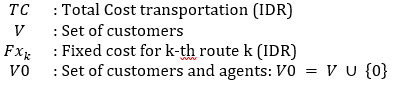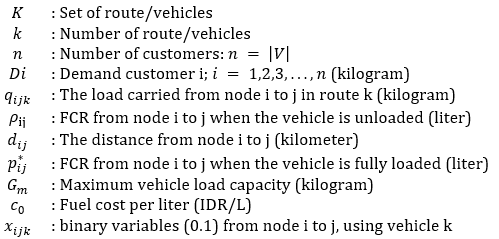The FCCVRP mathematical model was also developed from the method proposed by Xiao et al. (2012). It was developed by considering the node-dependent FCR. The objective function of the proposed model is formulated in Equation (1). Constraints of the proposed mathematical model are presented in Equations (2)-(7), with the model being developed as follows:

Objective Function: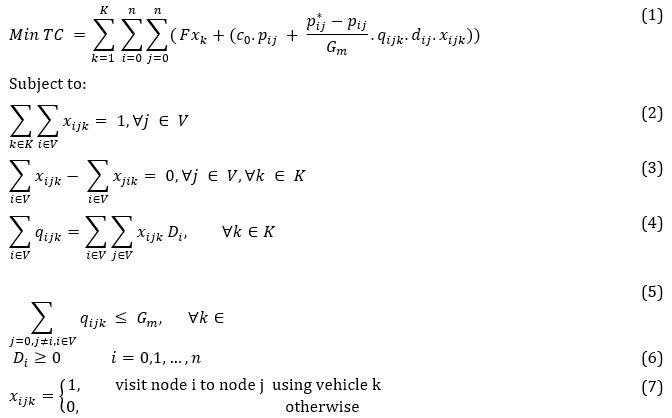Based on Equation (1), the reduction of the Total Cost (TC) was the objective function to be achieved in this study. This indicated that the fixed budgets (travel and fuel costs) were involved at the TC. Subsequently, the proposed fuel cost considered the nodal FCR, distance, price, and transported load quantity. The constraints of the FCCVRP mathematical model used were as follows: (i) Constraint (2) ensured that each customer was only visited by one vehicle, (ii) Constraint (3) indicated that vehicles should come and go from each customer, (iii) Constraint (4) showed that a load of all vehicles was equal to the demand from all customers, (iv) Constraint (5) stated that the loads did not exceed the maximum vehicle capacity, (v) Constraint (6) ensured that customer demand should not be negative, and (vi) Constraint (7) was the decision variable's binary number [0.1].

2.2. Hybrid Spotted Hyena Optimization (HSHO) Algorithm

This study proposed HSHO as a novel algorithm to solve the FCCVRP through the integration of the model and Neighborhood search implemented by Dhiman and Kumar (2017). This algorithm had five (5) main phases, namely (1) circling prey, (2) hunting, (3) attacking prey (exploitation), (4) searching for prey (exploration), and (5) neighborhood search. In the circling prey phase, the position of the spotted hyena was formulated in Equation (8), while a herd surrounding the prey was shown in Eqs. (9) and (10). This indicated that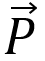and r = the position vector of the spotted hyena and a random number [0.1],the upper and lower bounds,the distance between the prey and spotted hyena,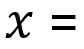the current iteration,the coefficient vectors, and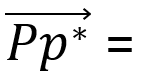the position vector of the prey. In addition,and  were calculated based on Eqs. (11)-(13), whereand  were random numbers [0.1].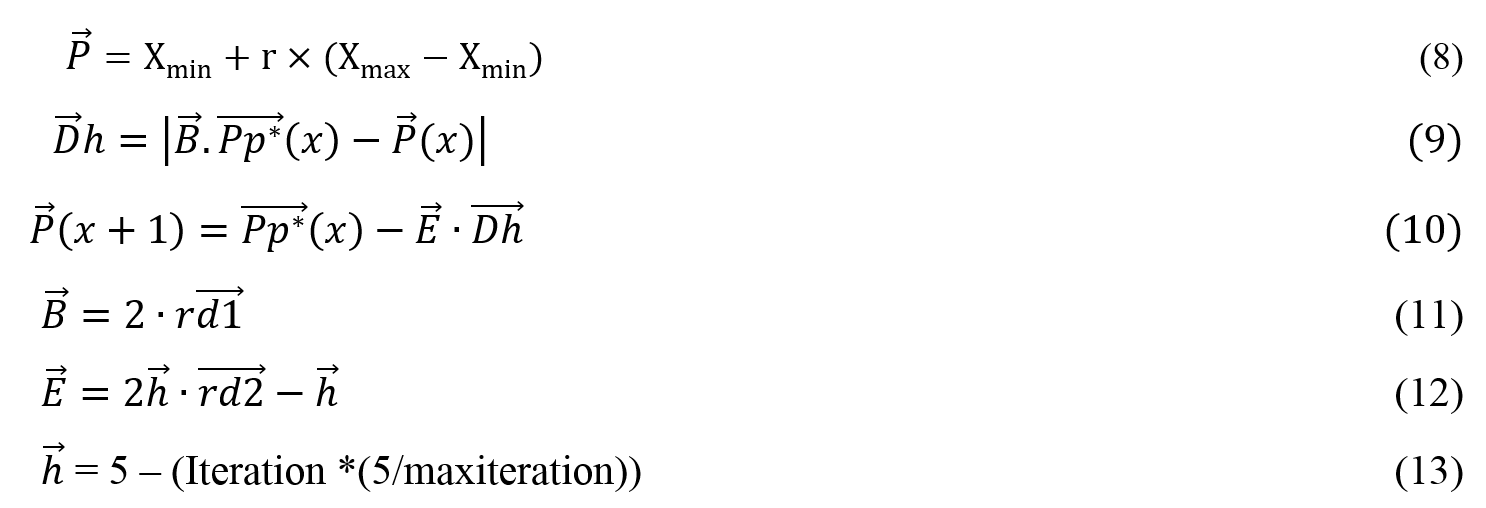According to the hunting phase, the best and optimal spotted hyenas had good knowledge (fitness) of prey locations. This showed that the herd created a cluster towards the best hyena, indicating the subsequent updates of their positions. These behaviors were modeled in Equations (14) to (16), where  = the position of the initial best hyena,  = the position of other hyenas, N = the number of spotted hyenas, calculated according to Equation (17), M = a random value (0, 5.1),  = the number of solutions after the addition of M, and  = the group or cluster of N-solutions.

Based on a discrete problem classified as a hard non-polynomial issue, the decision variable of FCCVRP was observed as the sequence of trips. This indicated the development of the Large Rank Value (LRV), to convert the position of the spotted hyena vector to the travel order. It is also an easy method to convert continuous values to the combinatorial problem, i.e., sorting from the largest to the smallest  (Utama and Widodo, 2021). The conversion of the spotted hyena position to the travel route is shown in Figure 1. In addition, the results of the trip sequence for each predator were used to calculate the total distribution cost in Equation (1).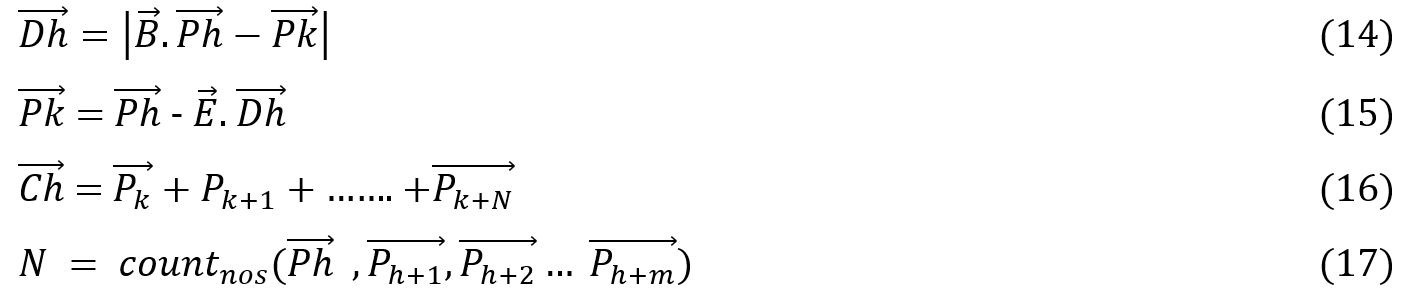In the attacking prey (exploitation) phase, the observations were modeled in Equation (18), wherewas determined as the new position of the spotted hyena. This indicated a decrease in the value of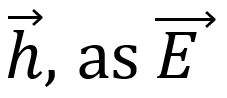changed with each additional iteration. It also showed that the herd moved away and closer to locate and attack the prey. When the value of |E|<1, E was subsequently generated from a variational random number [-1, 1]. In the searching phase (exploration), the spotted hyenas predictably moved away from the prey when the value of |E|>1.According to the neighborhood search phase, an exchange was applied to improve the algorithm performance in each iteration (Utama et al., 2020b). This indicated that two neighborhood exchange rules were proposed in this study, namely flip and swap, where a reversal was observed by transforming and exchanging two randomly selected position vectors of the spotted hyenas, as shown in Figures 2 and 3, respectively. The repetition of the neighborhood search was also suggested as 0.25 x the number of customers in each iteration. The LRV process was also applied as regards the conversion to a sequence of trips on each iteration. This was then compared with the previous processes to determine the best solution in the present iteration. In addition, algorithm 1 presented the complete Pseudo-code of the HHSO model, whose flow chart is shown in Figure 4.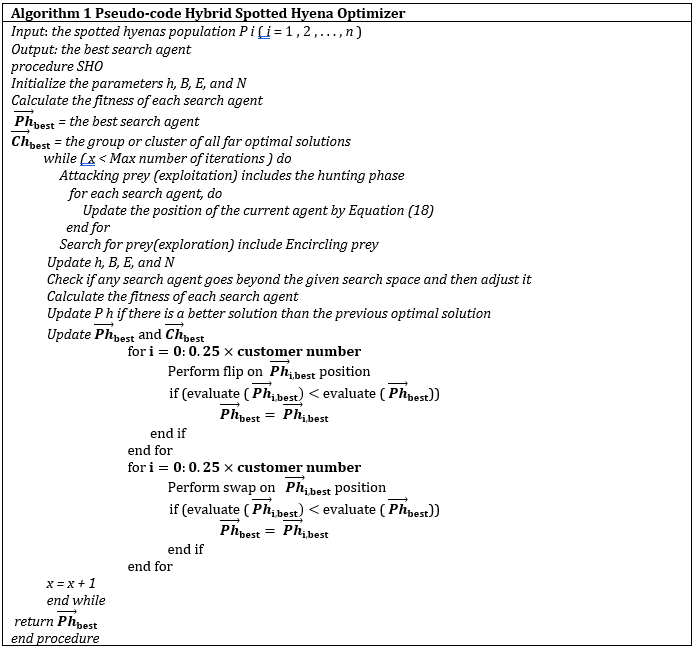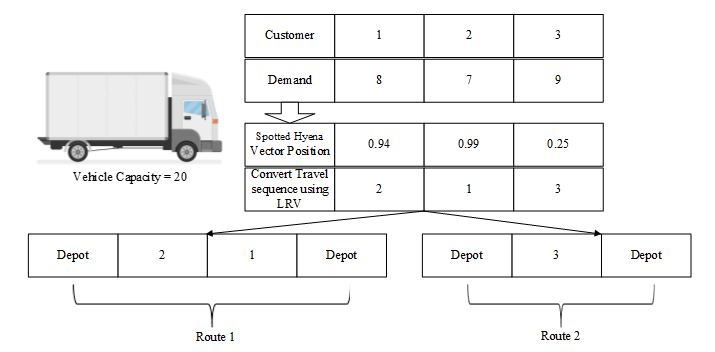Figure 1
The conversion of the spotted hyena's possition to the travel route

2.3.
Data collection and experiment setup

The data were obtained from the case study of a mineral water distribution company in Mojokerto, Indonesia, where the needs of 25 customers were to be met. The demand for each customer (Di) was between 379 and 905 kg, with the vehicle capacity (Gm) estimated to carry 2500 kg for one transport. The price of fuel per literwas also IDR 9,400, with the fixed cost for deliverybeing IDR 300,000. Furthermore, the company had 1 Distribution Centre (DC) for customer needs, as the distance observed between 1 DC and 25 consumers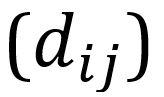was within 0.5-52 km. The FCRs from node i to j were also observed between 0.313-0.714 L/km and 0.625-1.429 L/km when the vehicle was unloaded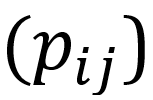and fully loadedrespectively. Based on the analysis, the iteration and population of HSHO were utilized with five variations each to test the parameters of this algorithm on the total cost of transportation. These five spotted hyena population parameters and HSHO iteration were applied between 50-500 and 10-200, respectively. In this test, approximately 25 trials were successfully conducted, with the best solution being stored for use in the sensitivity analyses. This test was subsequently used to examine the effect of the FCCVRP changes on the total, fixed, and fuel costs, respectively.

According to the sensitivity test, the transformed variables wereThis indicated that the five variations ofwere shifted from the initial values at each node. In cases 1/2 and 4/5, the initialvalue was decreased and increased by 0.1/0.05 and 1/1.5, respectively, with condition 3 completely utilizing this value. The initialvalues were also decreased and increased by 0.1/0.05 and 1/1.5 in cases 6/7 and 9/10, respectively, with condition 8 fully using this value. Infive data variations were applied with a value range of 8500 to 10500. Furthermore, five data variations were used forbetween 150000-400000, as comparisons with the state-of-the-art algorithms were applied to test the performance of HSHO. Based on the case study data, random parameters were generated forThis indicated the utilization of three variations, namely small (15 and 25 customers), medium (50 and 60 customers), and large (90 and 100 customers) cases, respectively. The utilized comparison algorithm was also SA (Kuo 2010), ACO (Yao et al., 2015), GA (Xiong, 2010), HPSO (Ali and Farida, 2021), Teaching–Learning-Based Optimization (TLBO) (Trachanatzi et al., 2021). These algorithms were then operated with 200 iterations and 500 population through the Matlab 2014a software on Windows 10 AMD A12 x64-64 8GB RAM processor.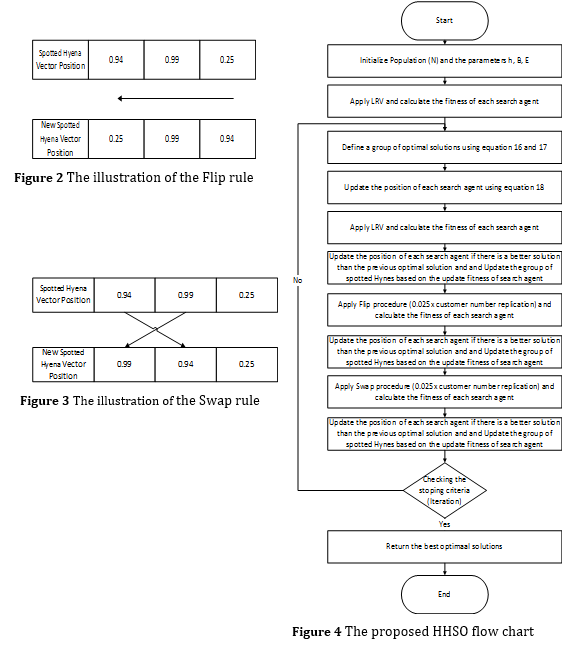Results and Discussion

3.1.  Effect of HSHO parameters on total transportation cost

The results of the HSHO effects on the total cost of transportation are presented in Table 1, where increasing iterations and population of the algorithm reduced the TC. However, decreased iterations and populations led to high total transportation costs. This indicated that large population parameters and HSHO interactions were needed to solve the FCCVRP. For a population of 500 and 200 iterations, the optimal result of TC was generated, as six routes were produced with a total transportation cost of 3,128,100 IDR.

Table 1 The effects of HSHO on total transportation cost (IDR)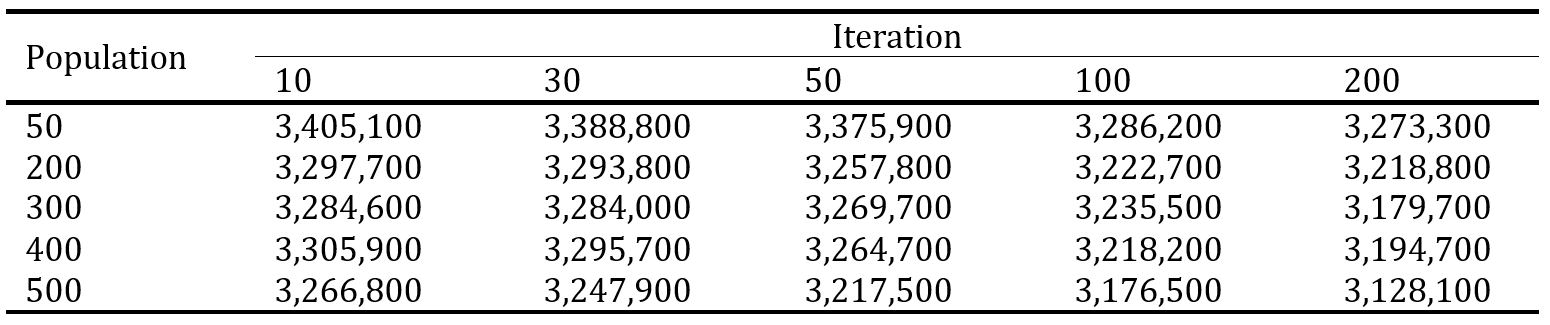3.2.  Sensitivity Analysis

The effects of thevalue changes towards the cost are shown in Tables 2 and 3, respectively, where both parameters significantly affected the total transportation budget. When the values ofwere increased and decreased, the total and fuel costs were also observed to be elevated and reduced, respectively. This indicated that the changes and the increase/decrease of both values significantly and insignificantly influenced the fuel and fixed costs, respectively. Therefore, the distribution company needs to minimize thevalues, to optimize the total transportation cost.

Table 2 The effect of thevalue change towards the cost (IDR)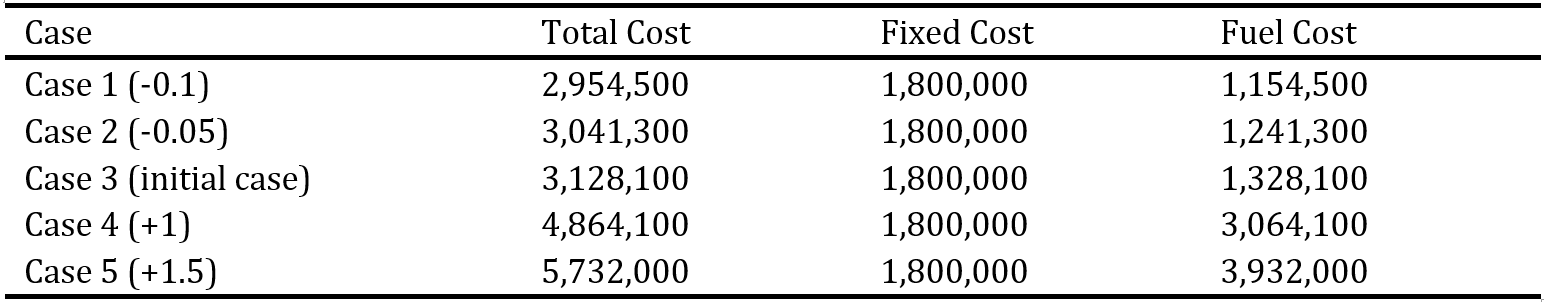Table 3 The effect of thevalue change towards the cost (IDR)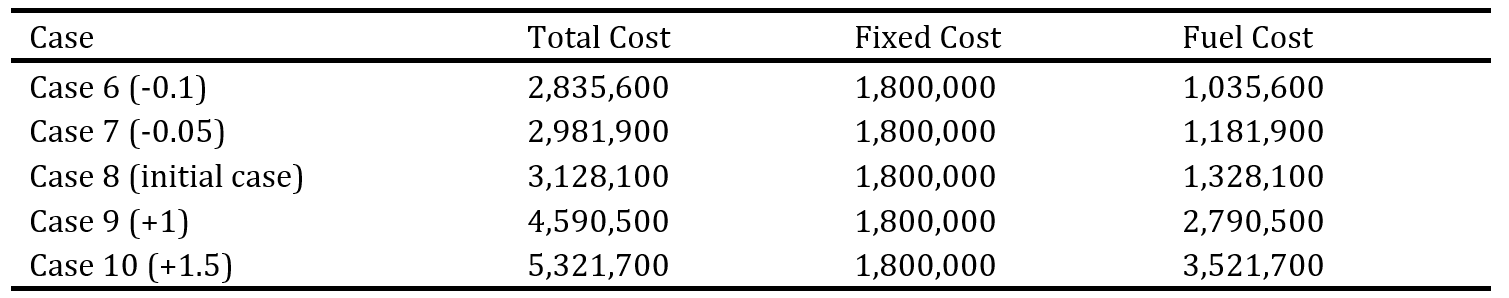The effect of thevalue change towards cost is presented in Table 4, where higher and lowerled to more expensive and cheaper fuel and total transportation costs, respectively. This indicated that thevalue had no significant effect on the fixed cost.

Table 4 The effect ofvalue change towards the cost (IDR)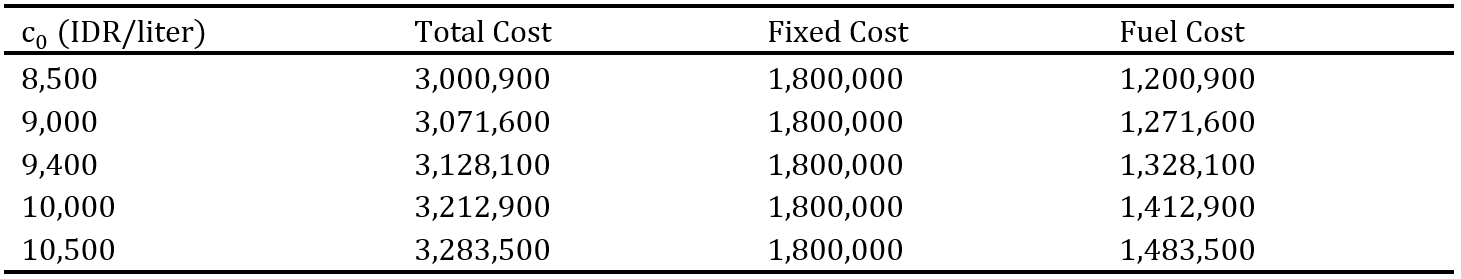The results of thevalue change towards the cost is shown in Table 5, where higher and lowervalues led to more expensive and cheaper transportation and fixed costs, respectively. This indicated that fuel cost had no significant effect on.

Table 5 The effect ofvalue change towards the cost (IDR)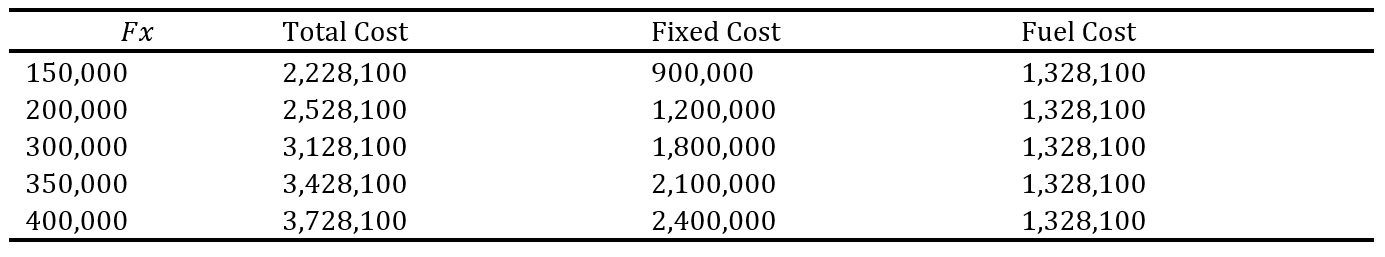3.3. Algorithm Comparison

Based on this study, a comparison of the HSHO algorithm was carried out against the SA, ACO, GA, HPSO, and TLBO algorithms. The comparative results towards the total transportation costs are shown in Table 6, where the proposed HSHO algorithm produced more optimal TC than the HPSO, TLBO GA, ACO, and SA algorithms. This was subsequently confirmed from the three variants of the trial case (small, medium, and large), which produced a better and optimal total transportation cost.

Table 6 Comparison of Algorithms to Total Transportation Costs (IDR)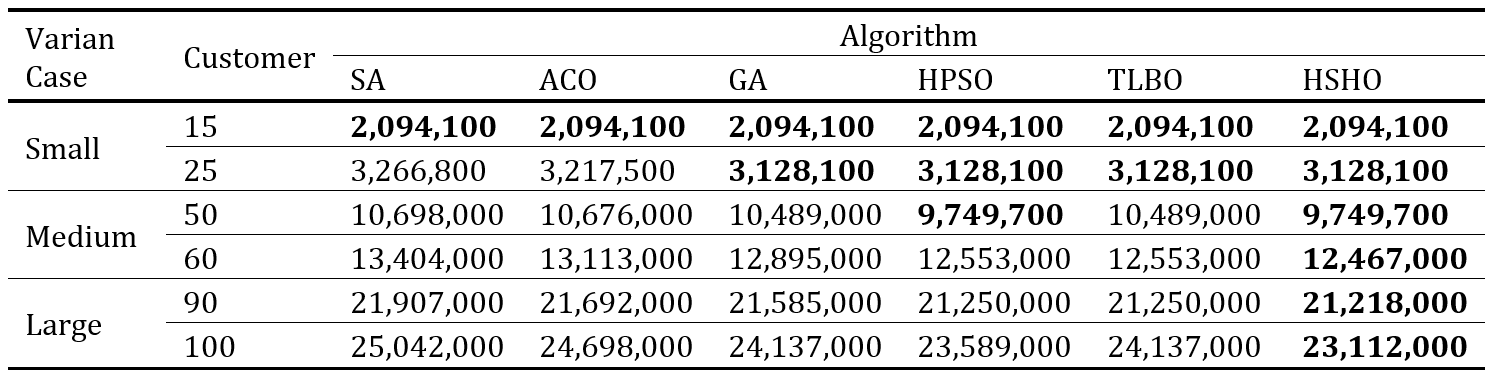Conclusion

This study presented the FCCVRP that considered the load-based inter-node FCR. In this condition, a new mathematical model and algorithm (FCCVRP and HSHO) were proposed as solution sources. Moreover, the HSHO was a combination of the SHO algorithm with the neighborhood search procedure. Based on the numerical experiments in the model, the changes observed inaffected the total transportation costs. This indicated that the increase in population parameters and HSHO iterations optimized the total transportation costs in the FCCVRP. The comparative analysis of algorithms also showed that HSHO produced optimal solutions in small, medium, and large cases compared to other algorithms. However, the proposed algorithm had limitations in considering a single distribution center, commodity delivery, and a homogeneous vehicle. Effective computation time was also ignored in solving the FCCVRP, indicating that future studies should consider multi-distribution centers, multi-commodity products, and vehicle heterogeneity. In addition, computation time should be considered in problem-solving.

Abdulameer, S.S., Yaacob, N.A., Ibrahim, Y.M., 2020. Measuring Leagile Supply Chain, Information Sharing, and Supply Chain Performance: Pre-Test and Pilot Test. International Journal of Technology, Volume 11(4), pp. 677687

Ali, M., Farida, B.N.I., 2021. Completion of FCVRP using Hybrid Particle Swarm Optimization Algorithm. Jurnal Teknik Industri, Volume 22(1), pp. 98112

Benyamin, A., Farhad, S.G., Saeid, B., 2021. Discrete Farmland Fertility Optimization Algorithm With Metropolis Acceptance Criterion For Traveling Salesman Problems. International Journal of Intelligent Systems, Volume 36(3), pp. 12701303

Deepradit, S., Ongkunaruk, P., Pisuchpen, R., 2020. Tactical Procurement Planning under Uncertainty in Aromatic Coconut Manufacturing. International Journal of Technology, Volume 11(4), pp. 698709

Dewi, S.K., Utama, D.M., 2021. A New Hybrid Whale Optimization Algorithm for Green Vehicle Routing Problem. Systems Science and Control Engineering, Volume 9(1), pp. 6172.

Dhiman, G., Kumar, V., 2017. Spotted Hyena Optimizer: A Novel Bio-Inspired Based Metaheuristic Technique for Engineering Applications. Advances in Engineering Software, Volume 114, pp. 4870

Gaur, D.R., Mudgal, A., Singh, R.R., 2013. Routing Vehicles to Minimize Fuel Consumption. Operations Research Letters, Volume 41(6), pp. 576580

Ghafori, S., Gharehchopogh, F.S., 2021. Advances in Spotted Hyena Optimizer: A Comprehensive Survey. Archives of Computational Methods in Engineering, Volume 2021, pp. 122

Ibrahim, M.F., Putri, M.M. Utama, D.M., 2020. A Literature Review On Reducing Carbon Emission From Supply Chain System: Drivers, Barriers, Performance Indicators, znd Practices. In: 3rd International Conference on Engineering Technology for Sustainable Development (ICET4SD) 23–24 October 2019, 2020 Yogyakarta, Indonesia, p. 012034

Kuo, Y., 2010. Using Simulated Annealing To Minimize Fuel Consumption For The Time-165dependent Vehicle Routing Problem. Computers and Industrial Engineering, Volume 59(1), pp. 157165

Naderipour, A., Abdul-Malek, Z., Hajivand, M., Seifabad, Z.M., Farsi, M.A., Nowdeh, S.A., Davoudkhani, I.F., 2021. Spotted Hyena Optimizer Algorithm for Capacitor Allocation in Radial Distribution System With Distributed Generation and Microgrid Operation Considering Different Load Types. Scientific Reports, Volume 11(1), pp. 115

Nguyen, V.D., Nguyen, T., Nguyen, T.L., Tran, V.C., Truong, H.B., 2020. Spotted Hyena Optimizer: An Approach to Travelling Salesman Problems. In: 12th International Conference, ICCCI, 2020 Da Nang, Vietnam, pp. 217228

Niu, Y., Yang, Z., Chen, P., Xiao, J., 2018. A Hybrid Tabu Search Algorithm for a Real-World Open Vehicle Routing Problem Involving Fuel Consumption Constraints. Complexity, pp. 112

Normasari, N.M.E., Yu, V.F., Bachtiyar, C., Sukoyo, 2019. A Simulated Annealing Heuristic for the Capacitated Green Vehicle Routing Problem. Mathematical Problems in Engineering, Volume 2019, p. 2358258

Norouzi, N., Sadegh-Amalnick, M., Tavakkoli-Moghaddam, R., 2017. Modified Particle Swarm Optimization In A Time-Dependent Vehicle Routing Problem: Minimizing Fuel Consumption. Optimization Letters, Volume 11(1), pp. 121134

Ozener, O., Ozkan, M., 2020. Fuel Consumption And Emission Evaluation of a Rapid Bus Transport System At Different Operating Conditions. Fuel, Volume 265, p, 117016

Poonthalir, G., Nadarajan, R., 2018. A Fuel Efficient Green Vehicle Routing Problem with Varying Speed Constraint (F-GVRP). Expert Systems with Applications, Volume 100, pp. 131144

Sahin, B., Yilmaz, H., Ust, Y., Guneri, A.F., Gulsun, B., 2009. An Approach For Analysing Transportation Costs and a Case Study. European Journal of Operational Research, Volume 193(1), pp. 111

Sahman, M.A., 2021. A Discrete Spotted Hyena Optimizer For Solving Distributed Job Shop Scheduling Problems. Applied Soft Computing, Volume 106, p. 107349

Sitompul, C., Horas, O.M., 2021. A Vehicle Routing Problem with Time Windows Subject to the Constraint of Vehicles and Good’s Dimensions. International Journal of Technology, Volume 12(4), pp. 865875

Suzuki, Y., 2011. A New Truck-Routing Approach for Reducing Fuel Consumption and Pollutants Emission. Transportation Research Part D: Transport and Environment, Volume 16(1), pp. 7377

Trachanatzi, D., Rigakis, M., Marinaki, M., Marinakis, Y., 2021. A Teaching–Learning-Based Optimization Algorithm For The Environmental Prize-Collecting Vehicle Routing Problem. Energy Systems, Volume 2021, pp. 128

Utama, D.M., Dewi, S.K., Wahid, A., Santoso, I., 2020a. The Vehicle Routing Problem For Perishable Goods: A Systematic Review. Cogent Engineering, Volume 7(1), p. 1816148

Utama, D.M., Farida, B.N.I., Fitriani, U., Ibrahim, M.F., Widodo, D.S., 2021a. Hybrid Henry Gas Solubility Optimization: An Effective Algorithm for Fuel Consumption Vehicle Routing Problem. Jurnal Ilmiah Teknik Industri, Volume 20(2), pp. 141152

Utama, D. M., Fitria, T.A., Garside, A.K., 2021b. Artificial Bee Colony Algorithm for Solving Green Vehicle Routing Problems with Time Windows. In: Virtual Conference on Engineering, Science and Technology (ViCEST) 2020, 2021/06/01 2021b Kuala Lumpur, Malaysia, p. 012043

Utama, D.M., Widodo, D.S., 2021. An Energy-Efficient Flow Shop Scheduling Using Hybrid Harris Hawks Optimization. Bulletin of Electrical Engineering and Informatics, Volume 10(3), pp. 11541163

Utama, D.M., Widodo, D.S., Ibrahim, M.F., Dewi, S.K., 2020b. An Effective Hybrid Ant Lion Algorithm To Minimize Mean Tardiness on Permutation Flow Shop Scheduling Problem. International Journal of Advances in Intelligent Informatics, Volume 6(1), pp. 2335

Utama, D.M., Widodo, D.S., Ibrahim, M.F., Dewi, S.K., 2020c. A New Hybrid Butterfly Optimization Algorithm for Green Vehicle Routing Problem. Journal of Advanced Transportation, Volume 2020, p. 8834502

Utama, D.M., Widodo, D.S., Wicaksono, W., Ardiansyah, L.R., 2019. A New Hybrid Metaheuristics Algorithm For Minimizing Energy Consumption In The Flow Shop Scheduling Problem. International Journal of Technology, Volume 10(2), pp. 320331

Xiao, Y., Zhao, Q., Kaku, I., Xu, Y., 2012. Development of a Fuel Consumption Optimization Model For The Capacitated Vehicle Routing Problem. Computers and Operations Research, Volume 39(7), pp. 14191431

Xiong, H., 2010. A Fuel Consumption Objective of VRP and the Genetic Algorithm. In: 2010 International Conference on E-Product E-Service and E-Entertainment, pp. 14

Yao, E., Lang, Z., Yang, Y., Zhang, Y., 2015. Vehicle Routing Problem Solution Considering Minimising Fuel Consumption. IET Intelligent Transport Systems, Volume 9(5), pp. 523529

Zhang, Z., Wei, L., Lim, A., 2015. An Evolutionary Local Search For The Capacitated Vehicle Routing Problem Minimizing Fuel Consumption Under Three-Dimensional Loading Constraints. Transportation Research Part B: Methodological, Volume 82, pp. 2035